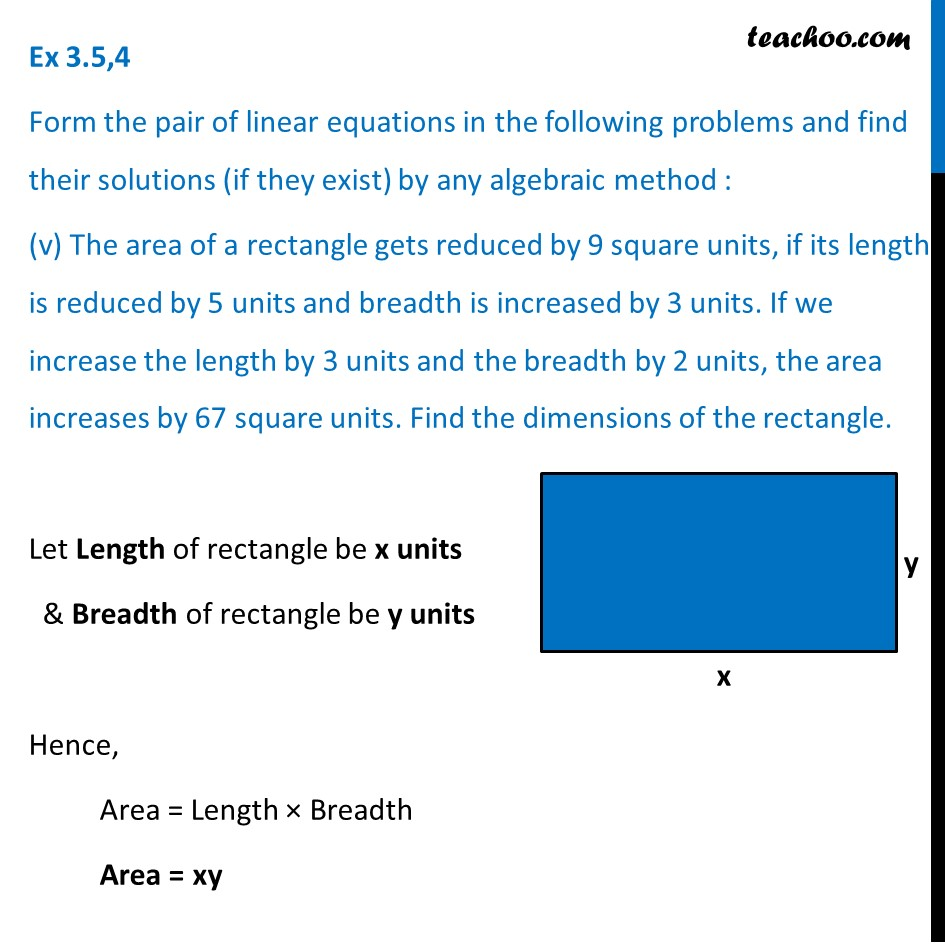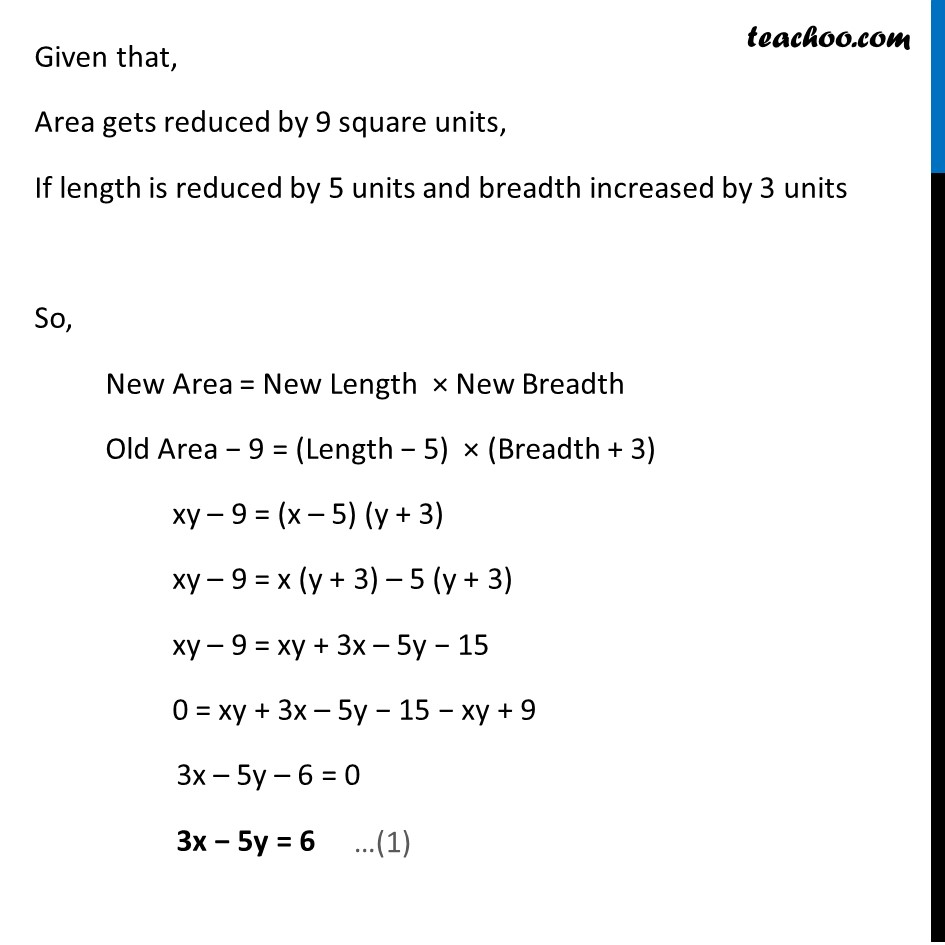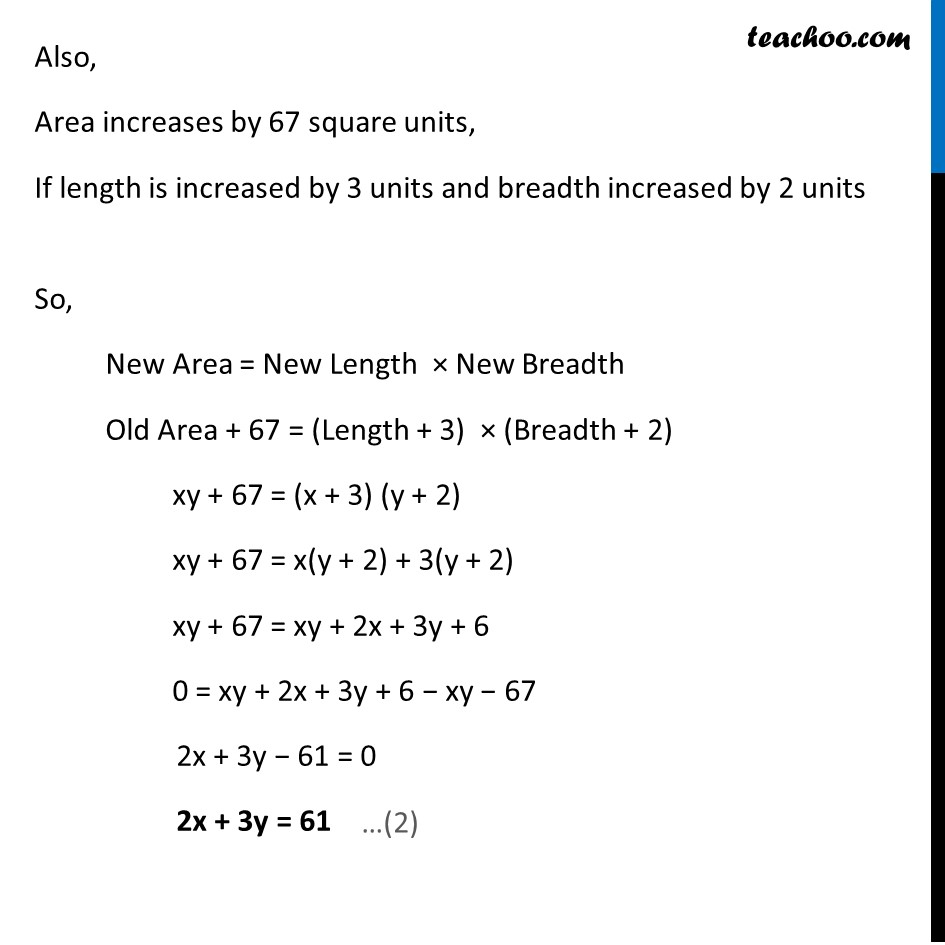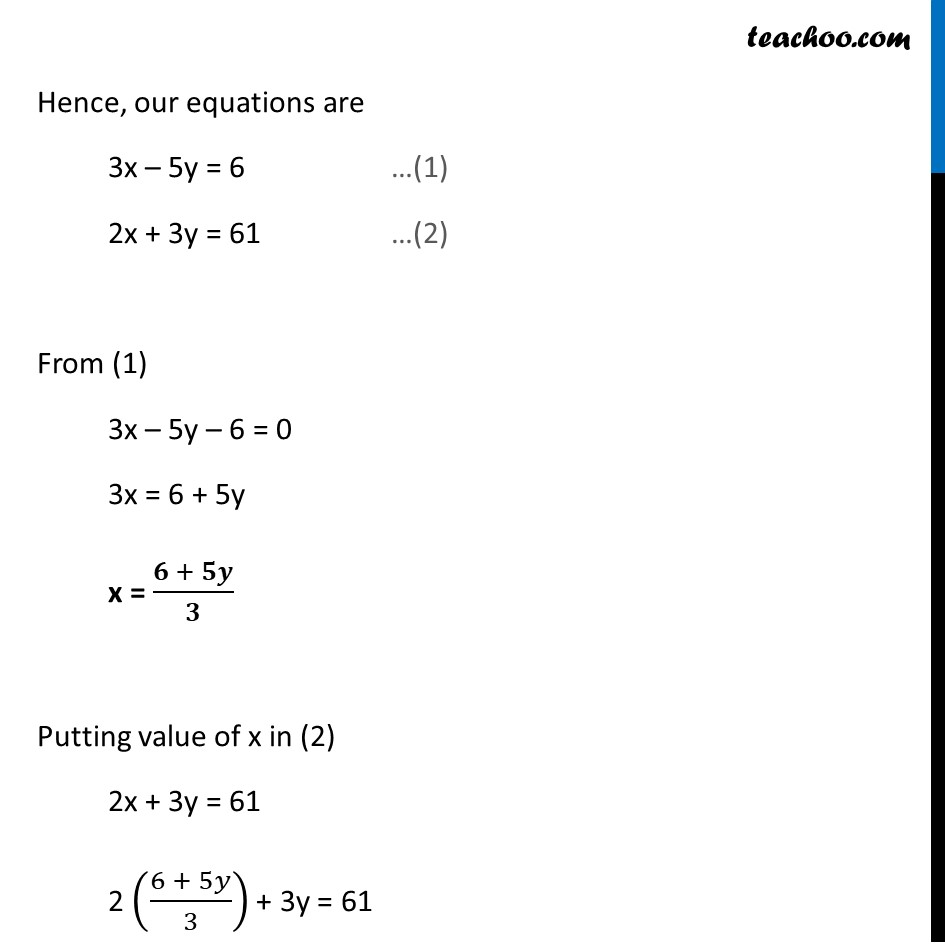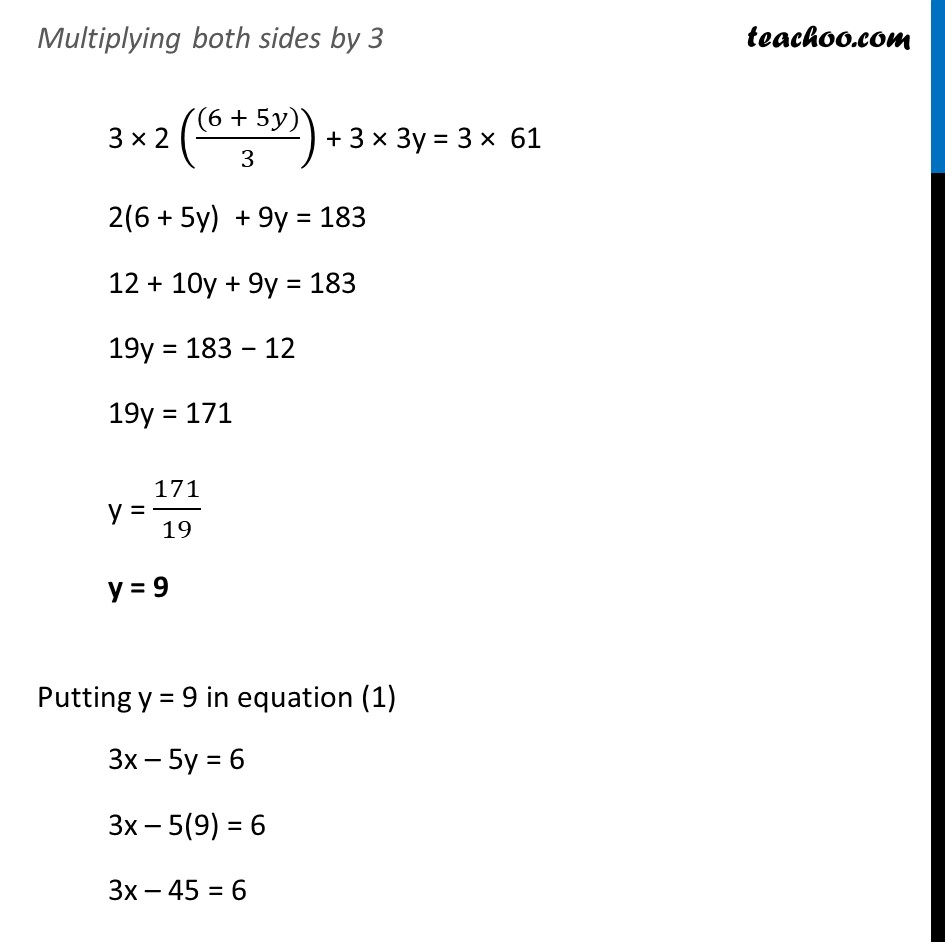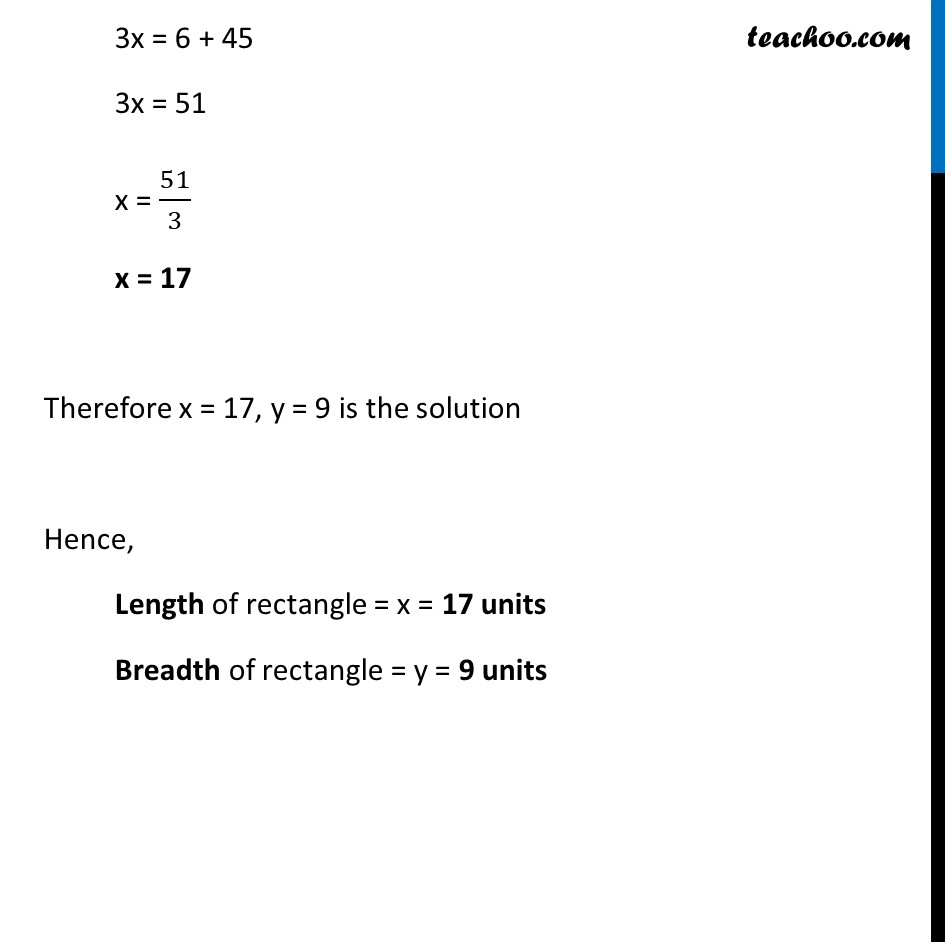1. Chapter 3 Class 10 Pair of Linear Equations in Two Variables (Term 1)
2. Serial order wise
3. Ex 3.5

Transcript

Ex 3.5,4 Form the pair of linear equations in the following problems and find their solutions (if they exist) by any algebraic method : (v) The area of a rectangle gets reduced by 9 square units, if its length is reduced by 5 units and breadth is increased by 3 units. If we increase the length by 3 units and the breadth by 2 units, the area increases by 67 square units. Find the dimensions of the rectangle. Let Length of rectangle be x units & Breadth of rectangle be y units Hence, Area = Length × Breadth Area = xy Given that, Area gets reduced by 9 square units, If length is reduced by 5 units and breadth increased by 3 units So, New Area = New Length × New Breadth Old Area − 9 = (Length − 5) × (Breadth + 3) xy – 9 = (x – 5) (y + 3) xy – 9 = x (y + 3) – 5 (y + 3) xy – 9 = xy + 3x – 5y − 15 0 = xy + 3x – 5y − 15 − xy + 9 3x – 5y – 6 = 0 3x − 5y = 6 Also, Area increases by 67 square units, If length is increased by 3 units and breadth increased by 2 units So, New Area = New Length × New Breadth Old Area + 67 = (Length + 3) × (Breadth + 2) xy + 67 = (x + 3) (y + 2) xy + 67 = x(y + 2) + 3(y + 2) xy + 67 = xy + 2x + 3y + 6 0 = xy + 2x + 3y + 6 − xy − 67 2x + 3y − 61 = 0 2x + 3y = 61 Hence, our equations are 3x – 5y = 6 …(1) 2x + 3y = 61 …(2) From (1) 3x – 5y – 6 = 0 3x = 6 + 5y x = (𝟔 + 𝟓𝒚)/𝟑 Putting value of x in (2) 2x + 3y = 61 2 ((6 + 5𝑦)/3) + 3y = 61 Multiplying both sides by 3 3 × 2 (((6 + 5𝑦))/3) + 3 × 3y = 3 × 61 2(6 + 5y) + 9y = 183 12 + 10y + 9y = 183 19y = 183 − 12 19y = 171 y = 171/19 y = 9 Putting y = 9 in equation (1) 3x – 5y = 6 3x – 5(9) = 6 3x – 45 = 6 3x = 6 + 45 3x = 51 x = 51/3 x = 17 Therefore x = 17, y = 9 is the solution Hence, Length of rectangle = x = 17 units Breadth of rectangle = y = 9 units